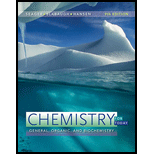Chapter 9, Problem 9.112EChemistry for Today: General, Orga...

9th Edition
Spencer L. Seager + 2 others
ISBN: 9781305960060

Solutions

Chapter
SectionChemistry for Today: General, Orga...

9th Edition
Spencer L. Seager + 2 others
ISBN: 9781305960060
Textbook Problem

Write equations similar to Equations 9.48 and 9.49 of the text to illustrate how a mixture of sodium hydrogen phosphate ( NaH 2 PO 4 ) and sodium dihydrogen phosphate ( Na 2 HPO 4 ) could function as a buffer when dissolved in water. Remember that phosphoric acid ( H 3 PO 4 ) ionizes in three steps.

Interpretation Introduction

Interpretation:

The equation that is similar to equations 9.48 and 9.49 to illustrate the way by a mixture of given compounds could act as a buffer on dissolution in water is to be stated.

Concept introduction:

A solution that consists of a mixture of a weak base and its conjugate acid, or vice versa is known as buffer solution. Buffer is a solution that has the ability to resist changes in pH when bases or acids are added.

The value of pH ranges from 0 to 14. Higher is the concentration of hydronium ion; lower is the value of pH.

Explanation

It is given that sodium hydrogen phosphate (Na2HPO4) and sodium dihydrogen phosphate (NaH2PO4) are the functions of a buffer solution.

The dissociation reaction of NaH2PO4 is as follows:

NaH2PO4Na++H2PO4Na2HPO42Na++HPO42

In the solution, H2PO4 and HPO42 are present as a buffering ions. The addition of acid to the solution involves the reaction of HPO42 ion with H+ results in the formation of H2PO4

Still sussing out bartleby?

Check out a sample textbook solution.

See a sample solution

The Solution to Your Study Problems

Bartleby provides explanations to thousands of textbook problems written by our experts, many with advanced degrees!

Get Started